Mars Size

Compare Mars and Earth using clay.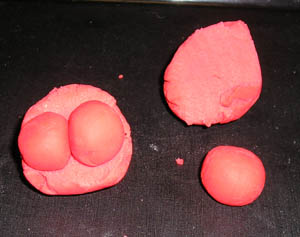Two Mars would fit across one earth.

Material

Clay or Play-Doh enough to make two, 5 cm diameter (2 inch), spheres

plastic knife

To Do and Notice

Make two, 5 cm diameter, spheres from the clay.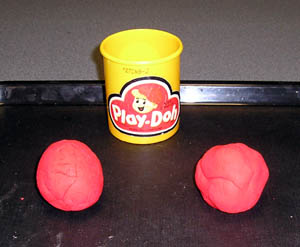Two 5 cm diameter spheres of Play-Doh.

Roll one of the spheres into a cylinder.

What fraction of the cylinder would you have to cut off to roll into a sphere that was 1/2 the diameter of the original sphere?

Cut the cylinder in half and roll it into a sphere.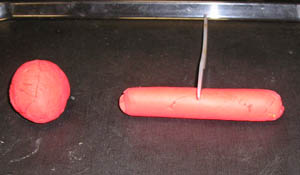Cut the cylinder in half.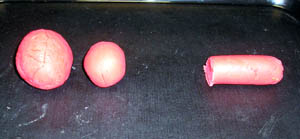And roll it into a sphere.

Notice that the resulting sphere has a diameter much greater than 1/2 the diameter of the earth sphere.

Cut the remaining 1/2 cylinder in half again, the resulting cylinder will have 1/4 the volume of the original sphere.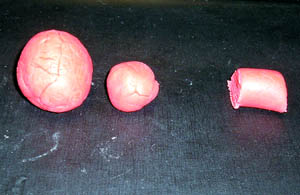Even 1/4 of the volume of the cylinder makes a sphere with a diameter larger than 1/2 that of the earth.

Finally cut the 1/4 cylinder in half again to make a sphere with 1/8 of the volume of the original earth. This will roll up into a sphere with 1/2 the diameter of the earth. This is easy to see if you cut the earth sphere in half and the mars sphere two then the two mars spheres will exactly fit across the earth sphere.A sphere with 1/8 the volume of the earth will have 1/2 the diameter of the earth.

What's Going On? Math Root.

The volume of a sphere, V, or radius R is given by the equation:

V = 4/3 pR3

So the volume of a sphere is proportional to the cube of the radius.

If the earth has a volume of 1, then Mars with 1/2 the diameter and so 1/2 the radius of the earth will have a volume of (1/2)3 = 1/8 of the earth.

Going Further

1. The volume of the earth's moon

The Moon has a radius of 1/4 of the earth.

Use the clay to find the volume of the original earth sphere needed to make a moon sphere with 1/4 the radius of the earth.

2. The surface area of Mars

How does the surface area of Mars compare to earth?

The equation for the area, A, of a sphere of radius, R , is:

A = 4pr2

So the area is proportional to the square of the radius.

The surface area of Mars is thus (1/2)2 = 1/4 the surface area of the earth.

Recall that 2/3 of the earth is covered by water, so that 1/3 of the earth is land. Thus the surface area of Mars is a little less than the entire land area of the earth.

Etc.

The mass of Mars is 1/10 the mass of the earth. Since the volume of Mars is 1/8 the volume of earth what does this mean?

Mars is less dense than earth. Mars could have a smaller iron core or the core could be partially made of elements other than nickel and iron. (The core of the earth is made of nickel iron.